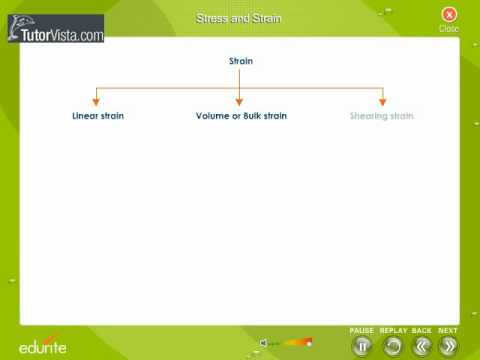### Main Difference

Many of you will be quite aware of Newton’s 1st Law of Motion even if you are not a science student. They were the basis of the scientific hit are being followed even today. Newton’s 1st Law of Motion states: “An object at rest stays at rest and an object in motion stays in motion with the same speed and in the same direction unless acted upon by an unbalanced force.” So with this one thing is clear that an external force is compulsion to make any change in an object or body. The force can even deform the object, stress and strain are interrelated and have a strong connection with the deforming forces. Stress is the measure of deforming force per unit area of the body, whereas strain is the relative change in the body’s length due to the deforming forces. Stress has the similar unit as of pressure, Pascal (Pa), whereas in strain where its all about change in length, it is denoted as the percentage wise change in length of the body due to deforming force.

### Comparison Chart

 Stress Strain Definition Stress is the measure of deforming force per unit area of the body. Strain is the relative change in the body’s length due to the deforming forces. Units Stress has the similar unit as of pressure, Pascal (Pa). In strain where its all about change in length, it is denoted as the percentage wise change in length of the body due to deforming force.

### What is Stress?

Stress is the measure of deforming force per unit area of the body. The stress on an object can change it physically or can even change its stability from rest to moving. Depending upon the direction, stress can be grouped into two main types, Normal Stress or Shear Stress. Shear stress is a stress state where the stress is parallel to the surface of the material, as opposed to normal stress when the stress is perpendicular to the surface. On the other hand, depending upon the physical change stress carries out to the objects it can mainly be grouped as Tensile Stress and Compressive Stress. The stress which led to increase in the length of objects it is called Tensile stress, whereas the stress compressing or shortening the length of object is called Compressive Stress.

Stress is denoted by σσ. It is expressed in N/m2 or Pa
Stress formula:Where,
F = Force applied
A = Area on which force is acting.

### What is Strain?

Strain is the measure of relative change in the body’s length due to the deforming forces. It is a dimensionless quantity, usually giving out the answers percentage-wise. Keeping it simple, the length before the deforming force brings out any change is noted therefore the change in length due to the deforming force afterwards is noted. The difference between both the measurements is the strain and it is expressed in terms of percentages. Strain can be mainly divided into three types, Longitudinal Strain, Shearing Strain and Volumetric Strain. Strain in a body is directly proportion to the stress.

Strain Formula:x = Change in dimension
L = Original dimension.

### Stress vs. Strain

• Stress is the measure of deforming force per unit area of the body, whereas strain is the relative change in the body’s length due to the deforming forces.
• Stress has the similar unit as of pressure, Pascal (Pa), whereas in strain where its all about change in length, it is denoted as the percentage wise change in length of the body due to deforming force.
• Strain in a body is directly proportion to the stress.